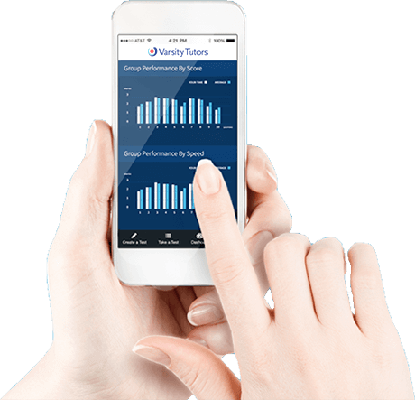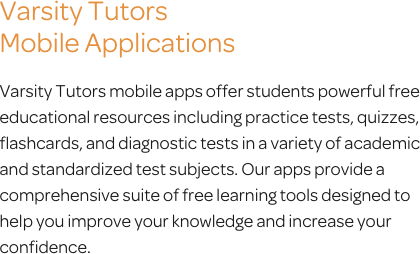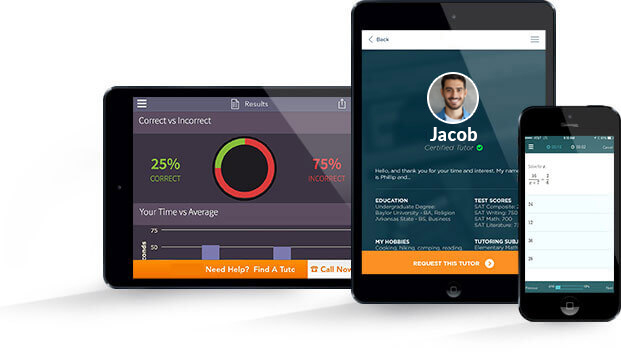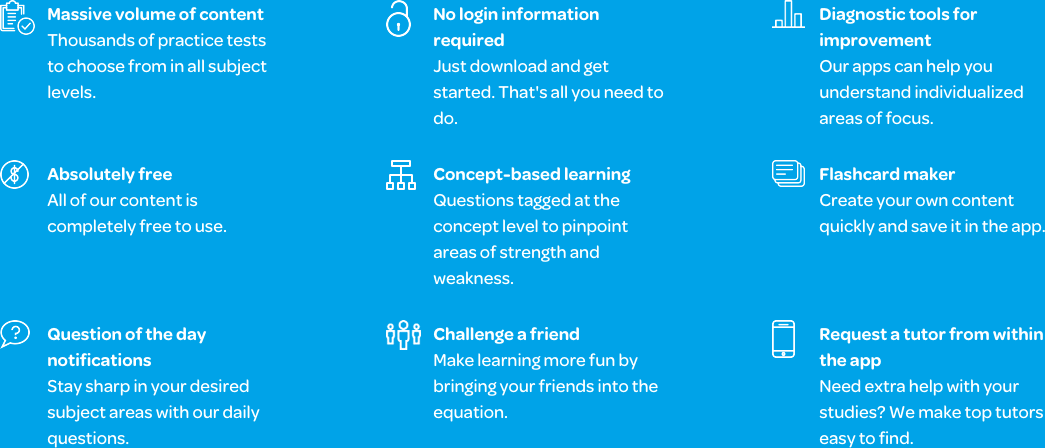# Varsity Tutors Calculus 2 mobile app### The Varsity Tutors Calculus 2 Mobile App

Calculus 2 is a course that increases upon the complexity of preceding math courses. It includes advanced math concepts such as derivatives, integrals, limits, and parametric and polar calculations. You’ll also encounter a power series, the sum of powers of a variable. Other topics include Lagrange Error, Euler’s method, and L’Hopital’s Rule, all of which the Varsity Tutors Calculus 2 app can help you master. This mobile device app is for iOS and Android devices and can be downloaded via iTunes or the Google Play Store.

Math problems in Calculus 2 require you to calculate arc length and exponential growth, use the ratio test to see if a series converges, or calculate the radius of convergence for a power series. When you are learning integrals, for example, it can be a challenge to master because you might not yet have applied them to the real world. During the course, you will find the area between curves, volume using cross sections, and volume of solids of revolution, problems which the disk and washer methods help to solve. Integrals also help you to analyze particle motion.

The Calculus 2 app provides several tools, such as practice tests, a Learn by Concept interactive syllabus, flashcards, and a convenient Question of the Day, that help you customize your study sessions to thoroughly master these subjects. The practice exercises from the different Learning Tools in the Calculus 2 app offer valuable practice with concepts such as derivatives, limits, functions, parametric, polar, and vector concepts. They also cover different types of series, including alternating, arithmetic/geometric series, and harmonic series.

Covering 40 questions each, the Full-Length Practice Tests give you a comprehensive review of the material, offering you the chance to solve problems based on the concepts you are required to master for the course. Running through the questions, you can quickly identify what you are comfortable with and which concepts you need to practice more. At the end, your score is displayed, along with the questions you got right and wrong. Your percentile and speed are shown, as if it were a real exam. Explanations to the right answers help make this process more effective, allowing you to fill in any gaps, whether the concept is understood or not.

Learn by Concept is another feature of the app. It lets you drill down to individual topics and sub-topics to find relevant questions. You can work on each problem and choose a multiple choice answer and then see the correct choice below the answer. Shorter concept-based practice tests function in a similar fashion as their longer counterparts, but they let you hone in on specific mathematical topics. You can also opt to work on the Question of the Day, which picks an item at random from the database of Calculus 2 material. The random content enables you to call on your knowledge and skills at a moment’s notice, which is also invaluable practice for this course. Lastly, flashcards give you quick reviews of one item at a time, and you can go through as many as you can do at once. Immediate answers and reviews also make these a convenient study method.

The Varsity Tutors Calculus 2 app for iPad, iPhone, and Android devices offers immediate access to these tools once you download it from the Google Play Store or iTunes. It can help take some of the stress out of your Calculus 2 course.Calculus can be intimidating even for those who love math, but it doesn't have to be. Students usually encounter Calculus 2 in college during their sophomore year. However, students who took Calculus 1 in high school may take Calculus 2 during their freshman year. It is recommended that regardless of which year one enrolls in Calculus 2, the classes are taken back to back so there is no loss of understanding between them. Calculus 2 may be a required course for several majors, including physics, economics, and several kinds of engineering. No matter your course, you can help yourself solidify the concepts of Calc 2 with Varsity Tutors’ Calculus 2 app, available for free download from iTunes and the Google Play Store.

In Calculus 1, students were introduced to the fundamental concepts of the field of calculus, which is simply the ways in which we are able to study change. Calculus 2 furthers this knowledge base by covering integrals and the applications of integrals, while also reviewing derivatives and limits. Parametric, polar, and vector functions are introduced and explored, as are sequences and series.

There is no single standardized exam for Calculus 2. Instead, the instructor usually evaluates student progress as they move through specific concepts during the duration of the course with periodic quizzes and tests. A cumulative midterm and final exam may be required, and likely will comprise a significant portion of the class grade. Because of the cumulative nature of the coursework, it is important for students to pay close attention to the feedback they receive on their work. If a concept is not fully mastered, it will become increasingly difficult to successfully move forward in the class. This is why comprehensive study tools, like those available from the free Varsity Tutors Calculus 2 app for iPhone, iPad, and Android devices, are an important supplement to your coursework.

Derivatives and limits were first explored in depth in Calculus I. Limit concepts will be reviewed to ensure students are able to find limits and understand the relationship between limits. You will also explore the relationship between limits and asymptotes, and limits and continuity. The definition of a derivative will be reviewed, along with the first and second derivatives of functions, and derivative at a point. New concepts involving derivatives will be introduced, including Euler’s Method and L’Hopital’s Rule.

Integrals were introduced in Calculus I, but not explored as fully as derivatives. In addition to reviewing Riemann Sums, the Fundamental Theorem of Calculus, and Integration Rules, new concepts will be introduced. You will learn how the process of integration relates to derivatives for Definite Integrals and Indefinite Integrals. The two types of Improper Integrals will be discussed, as well as the method of solving integrals by substitution. Integral applications in physics and other areas will be reviewed and enhanced.

Vector functions and equations are introduced in order to show students how to represent specific vectors in 2 and 3-dimensional space. A vector is used to show a specific quantity, such as force or velocity, that has both direction and magnitude. These vectors will be sketched in 2D to solve the magnitude and direction. You will also learn how to add, subtract, and multiply vectors, and to find the perpendicular vector. Finally, you will learn to evaluate the derivative, limit, and the integral of a 2D vector, as well as find the speed of a given vector.

Parametric functions and equations build upon the knowledge of vector functions and show how a vector can be rewritten in parametric form. Parametric equations of a curve show the coordinates of a curve as the function of a third variable known as a parameter. While the parameter is typically designated “t” for time, it may also represent another geometric variable or be used arbitrarily as a convenience. You will learn to understand the graph of a parametric equation and how to find the concavity and slope of a parameterized curve. The formula used to find the slope of a parametric function is later used to find the vertical and horizontal tangent lines of a parametric curve. Finally, you will learn to calculate the enclosed area of a parametric curve and find the length of a curve in parametric form.

Polar functions and equations are introduced with the concept of polar coordinates. Most students will be familiar with the Cartesian Coordinate System, which allows points to be plotted on vertical and horizontal distances along the x and y-axes. The Polar Coordinate System determines a point in the plane by using the distance from the origin, which is called a Pole, to the point on the x-axis, now called a Polar Axis. A new coordinate system is needed, because some situations are too difficult to implement in the Cartesian system. Most often these situations occur when dealing with spherical, circular, or cylindrical symmetry. While exploring this concept, you will learn to convert Polar and Cartesian coordinates, how to graph Polar Coordinates, and how to perform polar calculations.

Sequences and series are introduced, and you will learn to discuss them with terms such as divergence, convergence, monotonic, factorial, infinite, and bounded. Differences between a series and a sequence will be investigated, and you will learn to simplify factorials and to generate general terms. Specific series that will be explored include the Maclaurin Series, Power Series, Taylor Series, Alternating Series, Arithmetic Series, Geometric Series, and Harmonic Series. All in all, these topics require a lot of memorization and comprehension, but you can work to solidify both when you download the Varsity Tutors Calculus 2 app from iTunes or the Google Play Store.

By the end of this course, you will have a greater understanding of the complexities of calculus and be prepared to move on to Calculus 3. Once you begin to have a better understanding of these concepts, you will begin to see applications in your everyday life. It is important for students who are interested in pursuing higher levels of math and science not to let the reputation of calculus intimidate you into failure. Much of academic success is based on our expectations. If one expects calculus to be boring and difficult, it will become so. If one expects calculus will make it possible to see and understand broader mathematical principles in an enlightening and interesting way, that possibility will become a reality. You will need to take the time to thoroughly explore, practice, and play with each new concept to become familiar with it in order to gain mastery in the area and move on to the next concept. When you take your studying on the go with Varsity Tutors’ Calculus 2 app for your iPhone, iPad, or Android-powered device, you can give yourself the opportunity to adequately cover every topic you need to succeed in Calculus 2.